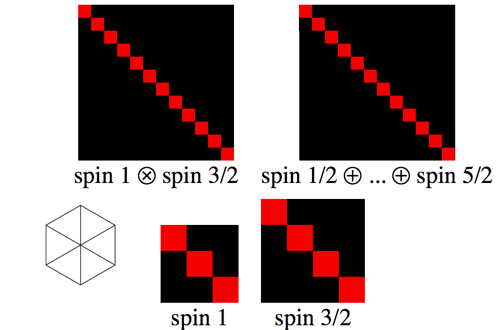Spin portrays the matrices of complex numbers that represent rotations in quantum mechanics.

Click on the applet to pause the animation, or redraw with two new random spins.

A quantum mechanical particle with a given amount of total spin, j, is described by a state vector in a complex vector space of dimension 2j+1. The two matrices in the bottom row of the diagram represent rotations for particles with two randomly chosen total spins, j1 and j2.

These matrices show the effect of the current rotation (portrayed in the inset) on a basis of vectors {|jm>}, m=–j, –j+1, ..., j–1, j, where m is the component of the particle’s spin measured in the direction of the z-axis. Successive columns of the matrix represent the rotated version of each of these vectors, with the brightness of the squares proportional to the magnitude of the vector’s complex coordinates, and the colour indicating their phase.

The top left-hand matrix shows the effect of the current rotation on the combined, two-particle system, which is described by state vectors in a complex vector space of dimension (2j1+1)(2j2+1). This matrix is constructed by taking each element of the j1 matrix, multiplying the entire j2 matrix by that value, and substituting the result in place of the original j1 element. The top right-hand matrix portrays the same transformation in a different basis, which is chosen to show how the two-particle state space can be split into a number of subspaces, each of which contains vectors that transform like single particles of definite spins, ranging from |j1j2| to j1+j2.Applets Gallery / Spin / created Sunday, 24 September 2000 / revised Sunday, 26 November 2000